Please visit, subscribe and share 10 Minutes Lectures in Computer Science

## Bigram Trigram and NGram in NLP, How to calculate the unigram, bigram, trigram, and ngram probabilities of a sentence? Maximum likelihood estimation to calculate the ngram probabilities

How to use N-gram model to estimate probability of a word sequence?
Let us consider Equation 1 again. For a Unigram model, how would we change the Equation 1?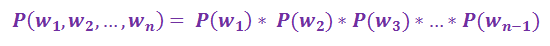Example: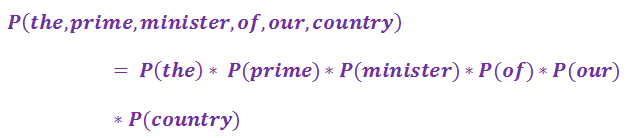For a bigram model, how would we change the Equation 1?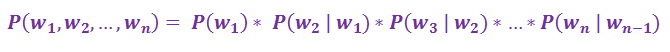Example: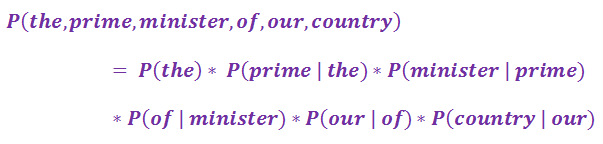For a trigram model, how would we change the Equation 1?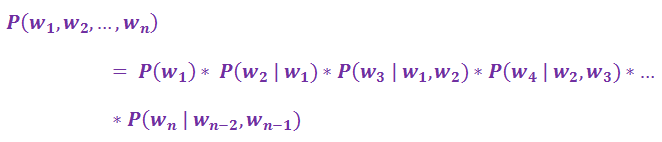Example: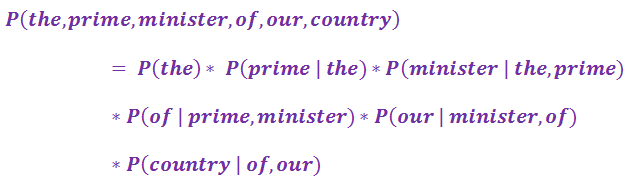Now, let us generalize the above examples of Unigram, Bigram, and Trigram calculation of a word sequence into equations.
Unigram: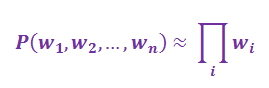Bigram: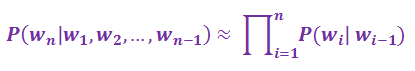In a Bigram model, for i=1, either the sentence start marker (<s>) or an empty string could be used as the word wi-1. [The empty string could be used as the start of the sentence or word sequence].
Trigram: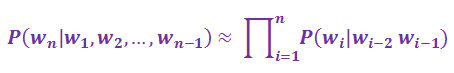In a Trigram model, for i=1 and i=2, two empty strings could be used as the word wi-1, wi-2. [The empty strings could be used as the start of every sentence or word sequence].

How do we estimate these N-gram probabilities?
We can use Maximum Likelihood Estimation to estimate the Bigram and Trigram probabilities. We get the MLE estimate for the parameters of an N-gram model by taking counts from a corpus, and normalizing them so they lie between 0 and 1.
For Bigram probability,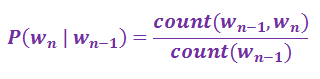Example: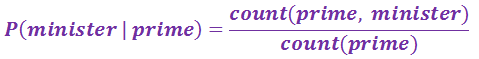The bigram probability is calculated by dividing the number of times the string “prime minister” appears in the given corpus by the total number of times the word “prime” appears in the same corpus.

For Trigram probability,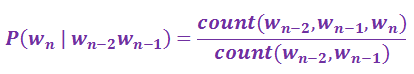Example: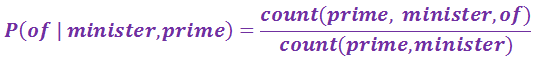The trigram probability is calculated by dividing the number of times the string “prime minister of” appears in the given corpus by the total number of times the string “prime minister” appears in the same corpus.

----------------------------------------------------------------------------------------------------------------------------